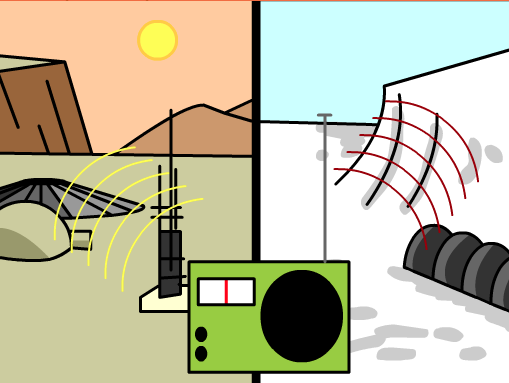SOUND
2 years ago
Save
Edit
Host a game
Live GameLive
Homework
Solo Practice
Practice15 QuestionsShow answers
• Question 1
30 secondsQ. Sound moves in a _________.
Transverse wave
Longitudinal wave
• Question 2
30 secondsQ. How is sound produced?
By the vibration of particles
By the transfer of particles
• Question 3
30 secondsQ. Area of a longitudinal wave where particles are CLOSE together.
Compression
Rarefaction
• Question 4
30 secondsQ. Area of a longitudinal wave where particles are APART.
Compression
Rarefaction
• Question 5
30 secondsQ. Which device measures sound waves?
Oscilloscope
Stethoscope
I don't know
• Question 6
30 secondsQ. The reflection of sound waves off of a hard surface can create ______.
A wave
An echo
• Question 7
30 secondsQ. Soft surfaces _______ sound waves.
Reflect
Absorb
• Question 8
30 secondsQ. ___________ measures  how close or  far particles are.
Amplitude
Frequency
• Question 9
30 secondsQ. ________ describes how LOUD a sound is.
Amplitude
Frequency
• Question 10
30 secondsQ. ____________ is determined by sound wave's frequency.
Compression
Pitch
• Question 11
30 secondsQ. _______ is the number of waves that pass a certain point at an specific time.
Frequency
Pitch
Amplitude
I don't know
• Question 12
30 secondsQ. ___________ affects how fast or slow sound waves travel.
Temperature
Speed
Amplitude
Frequency
• Question 13
30 secondsQ. Sound waves travel farther through ________ media, like water or steel.
Dense
Light
• Question 14
30 secondsQ. _______ is how high or low we listen/perceive  a sound.
Pitch
Frequency
Amplitude
I don't know
• Question 15
30 secondsQ. If you had to make some headphones to isolate sound, which material would you use?